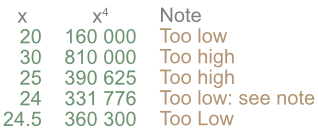Estimating Powers and Roots

# Estimating Powers and Roots

GCSE(H)

Estimating the power of a number involves rounding the number to one significant figure (1sf) and multiplying that number a suitable number of times.

Estimating the root of a number uses a technique known as Trial and Improvement. The calculation works by taking a guess at the answer. The root of a number is the inverse of taking the power. The guess of the answer is raised to that power and checked against the original number. The guess is revised, and the calculation repeated until sufficient accuracy is obtained.

## Examples

1. Estimate 4.4223.

2. Estimate the value of root(4)366218 to 2 significant figures.